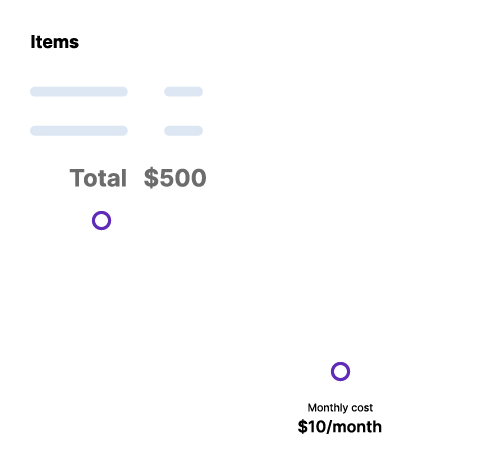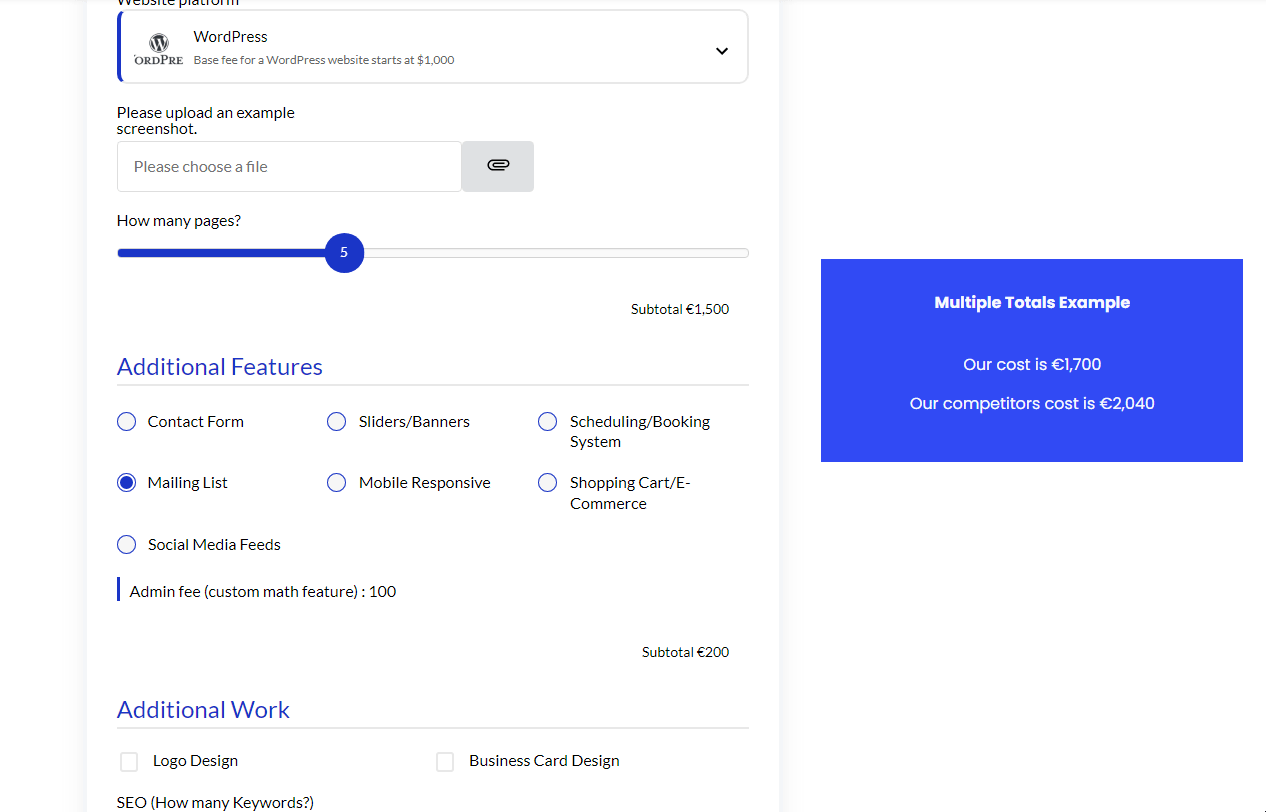# Custom Totals

A Complete GuideYou can place the calculator total anywhere on your website using a shortcode. You can either show the original total price or manipulate it with math for certain use cases (comparing competitive pricing and much more).

## Watch It In-Action

In this GIF example, we are showing what it would look like if you were to compare your competitor's pricing.## How to Activate & Use This Feature

Settings & Options

Enter the IDs of each calculator as we did below. In this example, we’re combining calculators #5 and #7

• Display the calculator total cost again somewhere else on the page

### Combine Calculators

Combine two calculator totals that are on the same page Enter the IDs of each calculator as we did below. In this example, we’re combining calculators #5 and #7

[scc_calculator-total combine='5,7']

### Currency Symbols

Use the currency-symbol attribute to disable the currency symbol. This attribute works if you use the ‘combine’ attribute.

[scc_calculator-total combine='5,7' currency-symbol=0]

### Apply Math

To apply math operations over the total value or the combined total value, you can use the ‘apply-math’ attribute.

[scc_calculator-total combine='5,7' currency-symbol=0 apply-math="divide:2"]

Here, the ‘divide:2’ is the math type, followed by the value the total amount should be divided against. You can use the multiply, add, and subtract operation over the value returned by the shortcode.
Here are the example.

[scc_calculator-total idvalue='2' apply-math="divide:2"]

(divides the value by 2)

[scc_calculator-total idvalue='2' apply-math="multiply:20"]

(multiplies the value by 20)

[scc_calculator-total combine='5,7' currency-symbol=0 apply-math="subtract:2"]

(combines two calculators & subtracts 23 from the value)

## Benefits, Features & Use Cases

Competitor Pricing – compare your pricing to a competitor’s pricing. A custom total shortcode should be added and multiplied by 1.15 if their prices are 15% higher than yours.

Show Monthly Pricing – calculate the monthly cost by dividing the total price by 12

Display the calculator total cost again somewhere else on the page. Use CSS code to style the way you want.

Combine two calculator totals that are on the same page.